# Reed's omega, delta, and chi conjecture

 Importance: High ✭✭✭
 Author(s): Reed, Bruce A.
 Subject: Graph Theory » Coloring » » Vertex coloring
 Keywords: coloring
 Posted by: mdevos on: May 22nd, 2007

For a graph, we define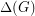to be the maximum degree,to be the size of the largest clique subgraph, and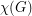to be the chromatic number of.

Conjecture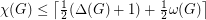for every graph.

Perhaps the two most trivial bounds onare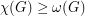and. The above conjecture roughly asserts that the (rounded-up) average of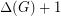andshould again be an upper bound on.

The conjecture is easy to verify whenis very large. It is trivial when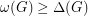, and it follows from Brook's theorem if. On the other hand, if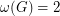, sois triangle free, then the conjecture is also true forsufficiently large. Indeed, Johannsen proved the much stronger fact that there exists a fixed constantso thatfor every triangle free graph.

Reed showed that the conjecture holds whenby way of matching theory. More interestingly, he proved (using probabilistc methods) that the conjecture is true provided thatis sufficiently large, andis sufficiently close to. More precisely, he proves the following:

Theorem   There exists a fixed constant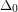such that for every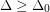, ifis a graph of maximum degreewith no clique of size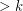for some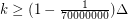then.

It is known that the conjecture is true fractionally (that is withreplaced by, the fractional chromatic number of~).

## Bibliography

*[R] B. Reed,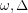, and, J. Graph Theory 27 (1998) 177-212.

* indicates original appearance(s) of problem.

### The statement of the

The statement of the conjecture is slightly incorrect. Instead of the +1 at the end, there should simply be a round-up. The conjecture is true for line graphs and quasi-line graphs, graphs with independence number 2, and any graph on I believe 12 vertices.

An outright proof of the result for triangle-free graphs would be very nice. Lovasz' result on splitting graphs is not quite enough in this case.

### changed

Thanks for the comment. I changed the statement to the conjectured (slightly stronger) version with round-up.